RUSSIAN JOURNAL OF EARTH SCIENCES, VOL. 20, ES3003, doi:10.2205/2020ES000712, 2020

# Petromagnetism and paleomagnetism of the Tarkhanian sediments in Kop-Takyl section (the Kerch Peninsula)

O. V. Pilipenko1,2, E. V. Filina2, Yu. V. Rostovtseva2,3, Z. Novruzov4

1Schmidt Institute of Physics of the Earth of RAS, Moscow, Russia

2Geophysical Center of RAS, Moscow, Russia

3Geological Faculty of the Lomonosov Moscow State University, Moscow, Russia

4Geological and Geophysical Institute of NASA, Baku, Azerbaijan

### Abstract

A petromagnetic and paleomagnetic study of the Tarkhanian sediments of the Kop-Takyl section was carried out in order to obtain data on magnetostratigraphy of the Miocene sedimentary succession of Eastern Paratethys. The Kop-Takyl section is located on the Black Sea coast of Kerch Peninsula and is composed mainly of clay deposits characterized by a weak magnetization. The main carriers of magnetization are a monoclinic pyrrhotite and a low concentration of magnetite. The total thickness of the studied deposits is $\sim 53-56$ m. Magnetic anisotropy was studied to justify the reliability of the obtained results. Paleomagnetic studies have shown that the considered interval of the Kuvinian beds of the Tarkhanian in the lower parts is composed of sediments of normal polyrity magnetization, and rocks in its upper part are reversed magnetized. The rocks of the Terskian and Argunian beds are characterized by normal polarity magnetization, too. For correct interpretation of the position of the Tarkhanian regional stage in the general stratigraphic scale, additional interdisciplinary research is required, including the study of these sediments by cyclostratigraphy methods to determine sedimentation rates and the possible duration of sediment accumulation.

### Introduction

The dating of the boundaries of the Miocene regional stages of Eastern Paratethys provokes sharp scientific discussions. The volume and stratigraphic position of the Tarkhanian regional stage in the Miocene in the general stratigraphic scale is controversial. This makes it difficult to carry out regional correlations and paleogeographic reconstructions.

As is known [Nevesskaya et al., 2004], the Tarkhanian is divided into three sub-stages – the lower (Kuvinian beds), middle (Terskian beds) and upper (Argunian beds). Currently, in the unified scale of the Miocene of Eastern Paratethys, the lower part (Kuvinian and Terskian beds) of the Tarkhanian is related to the Lower Miocene, and the upper part (Argunian beds) – to the Middle Miocene [Nevesskaya et al., 2004]. According to V. M. Trubikhin [Trubikhin, 1998], the base of the Tarkhanian approximately coincides with the base of the Middle Miocene Langhian of the Mediterranean stratigraphic scale, and according to D. Palcu [Palcu et al., 2019] it corresponds to its middle part.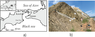Figure 1

The Tarkhanian sediments of the Kop-Takyl section in Kerch Peninsula of the Black Sea coast, are an important object for study, since they are represented by relatively deep-water clays, characterized by a greater completeness of the geological record compared to shallow sediments. These sediments are unexplored in paleomagnetic terms, compared with the Tarkhanian succession of the Maly Kamyshlak neostratotypic section, located on the Azov coast of the Kerch Peninsula (Figure 1a).

This research deals with a petromagnetic and paleomagnetic study of the sediments of the Kop-Takyl section, in order to obtain the new paleomagnetic and magnetostratigraphic data on the Tarkhanian regional stage of the Eastern Paratethys of Miocene. These results are also essential for analysis of the studied sediments by cyclostratigraphic methods.

### Object of Research

The Kop-Takyl section is located on the Black Sea coast of Kerch Peninsula ($\varphi=45\mbox{°} 10'$ N, $\lambda=36\mbox{°} 44'$ E), (Figure 1a), south to the settlement of Zavetnoe and is composed mainly of clays containing carbonate layers. The Tarkhanian sediments of the Kop-Takyl section form the northern flank of the anticline, Figure 1b.

A carbonate layer of $\sim 0.2$ m is located in the lower part of the section Kop-Takyl, which corresponds to Terskian beds and is known in literature as the "Tarkhanian marlstone" [Nevesskaya et al., 2004]. This layer is a reliable lithological marker (Figure 1b, layer 2) and contains a marine fauna: Abra parabilis, Lentipecten corneus denudatus, Abra alba etc. [Golovina and Goncharova, 2004].

Sediments located under the Tarkhanian marlstone belong to the Kuvinian beds (Figure 1b, Layer 1) [Golovina and Goncharova, 2004]. Rocks lying above the Tarkhanian marlstone are corresponded to the Argunian beds (Figure 1b, Layers 3–4), [Golovina and Goncharova, 2004].

The Argunian beds are predominantly represented by clays and are characterized by an abundance of spiratellas (spirialis clays) and depletion of the remaining groups of marine fauna. All this is evidence of a more difficult connection between the existing basin and open sea waters [Goncharova, 1989]. In the lower part of the Argunian beds, in the Tarkhanian neostratotype in Kerch Peninsula, the boundary of nannoplankton zones NN4-NN5 is distinguished, which may be the level of comparison of magnetic zones [Molostovsky and Khramov, 1997].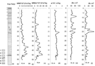Figure 2

The studied sediments in the Kop-Takyl section are subdivided on next layers upwards (Figure 2a):

1. Dark gray (up to black) clays, laminated, with jarosite, with a landslide at the base: $\sim 4$ m.
2. Light grey carbonate layer ("Tarkhanian marlstone"), fine-grained, lenticular, with mollusk shells Lentipecten corneus denudatus: $\sim 0.2$ m.
3. Dark grey clays, laminated, with local pyritization, calcareous at the base (3–4 m), with rare mollusk shells, with thin sandy interlayers in the middle part, with separate carbonate nodules and layers in the top: $\sim 33-34$ m.
4. Grey-green clays, laminated, with beds of carbonate rocks, in the upper part with an abundance of pteropod shells (spiratella): $\sim 10–11$ m.
5. Interbedding of grey-green laminated clays with carbonate rocks: $\sim 5-6$ m.
6. Interbedding of carbonate rocks, carbonates with signs of local secondary dolomitization: $\sim 1-1.2$ m.

The total thickness of the deposits is $\sim 53-56$ m. In the Kop-Takyl section, the average elements of bedding of the Tarkhanian rocks are: bearing azimuth $Az=328\mbox{°}$, incidence angle $\angle = 48\mbox{°}$. The studied Tarkhanian sediments as a whole have a similar lithology with analogues sedimentary successions of the Maly Kamyshlak and Skelya sections located on the Azov coast of the Kerch Peninsula [Popov et al., 1996; Rostovtseva, 2012].

### Selection of Samples for Petromagnetic and Paleomagnetic Research

For petromagnetic and paleomagnetic studies, hand blocks (44 pcs.) of rocks were collected from the Lower Tarkhanian (Kuvinian beds) base to the upper part of the Upper Tarkhanian (Argunian beds) sediments with an interval of $\sim 1.5$ m. Oriented with respect to the magnetic meridian hand blocks were taken mainly from freshly cleaned vertical sections. Further, the hand blocks were sawn up into horizontal plates, from which in their turn oriented cubic samples were made with an edge of 2 cm, 2–3 samples per level. The total number of studied samples was 125. To conduct thermomagnetic analyzes, samples were cut into cubes with a 1 cm edge, two samples per level.

### Equipment and Methods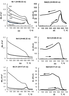Figure 3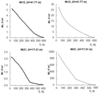Figure 4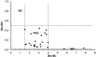Figure 5

The standard magnetic parameters were measured and investigated: the natural remanent magnetization NRM, the magnetic susceptibility {æ}, the magnetic coercitivity force $Bc$ and the remanent magnetic coercitivity force $Bcr$, (Figure 2). Three types of thermomagnetic analysis were fullfiled: according to the temperature dependence of the saturation magnetic moment $Ms (T)$ (Figure 3), the magnetic susceptibility ${æ} (T)$ (Figure 4), and the remanent magnetic moment $Mr (T)$ (Figure 5). The whole experiment was carried out in the Laboratory of Main Geomagnetic Field and Rock Magnetism of Schmidt Institute of Physics of the Earth RAS. NRM measurements were carried out with a JR-6 magnetometer (AGICO, Czech Republic, the sensitivity of the device is $2\times 10^{-6}$ A/m) and a SQUID magnetometer (2G Enterprises, USA, the sensitivity of the device is about $10^{-7}$ A/m). {æ}, an anisotropy of magnetic susceptibility AMS, and thermomagnetic analysis ${æ} (T)$ were fulfilled with a Multi-Function Kappabridge MFK1-FA with CS3 attachment (AGICO, Czech Republic). $Bc$, $Bcr$, $Mr$ and $Mrs$ were measured on pieces of rock from all collected levels with a VSM vibromagnetometer (PMC Micro Mag 3900, USA). Thermomagnetic analysis $Ms (T)$ was carried out with a vibromagnetometer designed by Yu. K. Vinogradov (Orion, Russia), thermomagnetic analysis $Mr (T)$ – with a three-component thermomagnetometer (Orion, Russia). The alternating field demagnetization was carried out by a device of Applied Physics Systems (USA) in three positions of the sample inside an alternating current coil, the magnetic field of which could vary from 0 to 100 mT with a shielded external magnetic field, as well as in a SQUID magnetometer (2G Enterprises, USA), the magnetic field of which could vary from 0 to 130 mT. SQUID magnetometer was placed in a non-magnetic room (Lodestar Magnetics, USA), which shields the samples from exposure to external magnetic fields. All samples were weighed on a CAUY120 balance (CAS-electronic balance, Japan) before the study has started.

### Rock Magnetic Properties

Before the AF demagnetization NRM was measured and normalized to sample density. The NRM value for the series of the first duplicate samples is shown in Figure 2b. The average NRM value for three samples from the level for the studied part of the section is divided into two parts. In the lower part of the section, at depths from 55–34 m, the NRM value is heterogeneous and varies by $\sim 5$ times in the range $(0.12-0.57)\times 10^{-6}$ A m$^2$/kg. In the upper part of the section, at depths from 2–34 m, the NRM value has lower values $(0.05-0.28)\times 10^{-6}$ A m$^2$/kg, is also heterogeneous and varies by $\sim 6$ times.

The {æ} values are low, varying along the section from $0.67 \times 10^{-7}$ to $1.47 \times 10^{-7}$ m$^3$/kg, which gives a rough estimate of the change in the concentration of carrier particles of natural remanent magnetization along the section by $\sim 2.2$ times [Pan et al., 2001]. Changes in {æ} correspond to NRM variations in the section (Figure 2b, Figure 2d), and demonstrate an increase in magnetic susceptibility with depth, which reflects an increase in the concentration of magnetic minerals in sediments from top to bottom in the section [Tarling and Hrouda, 1993]. The linear correlation coefficient between NRM series and {æ} before demagnetization is highly significant: $r = 0.63$ for the pairs of points involved in the comparison $N = 44$ [Taylor, 1985]. A highly significant correlation means that the value of the NRM depends on the concentration of the main carriers of the natural remanent magnetization.

The magnetic coercitive force $Bc$ varies in the range of 5–35 mT, the remanent magnetic coercive force $Bc$ varies in the range of 28–64 mT (Figure 2f, Figure 2e), with the exception of three samples. The measured and calculated value of the coercitive ratio $Mrs/Ms$ for the studied part of the section varies in the range 0.02–0.44, with the exception of three samples too. Thus, the main carrier of magnetization in these rocks is a low-coercitive magnetic mineral.

With a goal to study the composition of magnetic minerals – carriers of natural remanent magnetization, a pilot collection of the samples was selected, consisting of 10 samples (two doubles), volume $\sim 1$ cm$^3$: N 3 ($H=52.12$ m), 4–2 ($H =50.62$ m), 8 ($H=46.22$ m), 12 ($H=41.77$ m), 35($H=31.94$ m), 39 ($H=27.20$ m), 18 ($H=21.52$ m), 21 ($H=17.41$ m), 25 ($H=12.11$ m), 30 ($H=4.74$ m). Three types of thermomagnetic analysis were performed: 1) with a vibromagnetometer designed by Yu. K. Vinogradov (Orion, Russia) according to the dependence $Ms(T)$ in a constant magnetic field of $0.7 T$; 2) with Multi-Function Kappabridge (AGICO, Czech Republic) by ${æ} (T)$ on powder samples weighing $\sim 1$ g; 3) according to the temperature dependence of the remanent magnetic moment created in the magnetic field of $0.7 T$.

Thermomagnetic analysis of the temperature dependence of the saturation partial magnetic moment created in a field of $0.7 T$ (Figure 3a) showed that up to 450° C the $Ms (T)$ curves are paramagnetic and reversible. After 450° C, an increase of the magnetic moment is observed, the curves cease to be reversible. After heating up to 600° C, an inflection point appears in the first heating curve. This point does not shift during further heating and correspond to the magnetite Curie temperature.

Figure 3b, Figure 3c shows the results of thermomagnetic analysis of $Ms (T)$ in a field of $0.7 T$. The $Ms (T)$ curves of the first heating of the samples also have a paramagnetic form. After $\sim 450$° C, an increase of the magnitude of the magnetic moment is often observed. Even at temperatures of 700° C the magnetic moment is not completely destroyed, and the paramagnetic component remains. The curves of the second heating pass much higher than the curves of the first heating and have an inflection at a temperature of $\sim 580$° C, which indicates the formation of magnetite during heating. An increase in the magnetic moment after 450° C is possibly associated with the formation of magnetite from pyrite.

Thermomagnetic analysis ${æ} (T)$ of powdery rock samples from the same stratigraphic levels of the Kop-Takyl section was carried out (Figure 3d, Figure 3e, Figure 3f) with the Multi-Function Kappabridge cappameter (AGICO, Czech Republic). This type of thermomagnetic analysis showed an increase in magnetic susceptibility after $\sim 300$° C (Figure 3d, Figure 3f), and the presence of a Curie point for magnetite both on the heating curve and the cooling curve, which indicates the formation of magnetite from non-magnetic minerals (e.g. pyrite).

Thermomagnetic analysis was carried out with a three-component thermomagnetometer, according to the temperature dependence of the remanent magnetic moment $Mr$ created in a field of $0.7 T$, of clay samples with a volume of 1 cm$^3$ with sufficiently high initial values of NRM (NN 1 ($H=55.32$ m), 8 ($H=46.22$ m), 12 ($H=41.77$ m), 21 ($H=17.41$ m)). The analysis of other samples was not possible because of the low initial NRM values and, as a consequence, the low values of the remanent magnetic moment.

Thermomagnetic analysis for sample N 1 showed that the curves of the remanent magnetic moment $Mr (T)$ of the first heating have inflection points at $\sim 320$° C and $\sim 450$° C. $Mr$ is completely destroyed at $\sim 550$° C. The second heating curves extend much higher than the first heating curves and have an inflection at a temperature of $\sim 580$° C, which indicates the formation of magnetite during heating. Thus, in this sample, there may be monoclinic pyrrhotite with a blocking temperature of $\sim 320$° C and magnetite with a blocking temperature of $\sim 550$° C. The inflection at $\sim 450$° C and the strong increase in the magnetic moment in the second heating curve are most likely associated with the formation of magnetite from pyrite.

Thermomagnetic analysis of sample N 12 showed that the $Mr (T)$ curves of the first heating have a paramagnetic form with an inflection point at $\sim 575$° C, Figure 4. The second heating curve extends well above the first one. The magnetic moment is completely destroyed at a blocking temperature of   575° C. Thus, the main magnetic carrier of magnetization in this sample is a low concentration of magnetite. The increase in the magnetic moment after heating is explained by the formation of new magnetic grains of magnetite from non-magnetic compounds.

Thermomagnetic analysis of sample N 21 showed that the $Mr (T)$ curves of the first heating have a convex shape with an inflection point at $\sim 360$° C and 560° C, Figure 4. Even at a temperature of 600° C the magnetic moment does not completely break down and the paramagnetic component remains. The second heating curve passes much higher than the first heating curve, the magnetic moment is completely destroyed at a blocking temperature of $\sim 560$° C. On the second heating curve, there is an inflection point at $\sim 300$° C. Thus, the main carriers of magnetization in this sample are monoclinic pyrrhotite and magnetit, possibly. The increase in the magnetic moment after heating is explained by the formation of new magnetic grains of magnetite from non-magnetic compounds, for example, pyrite.

Thus, magnetite and monoclinic pyrrhotite are probably the main magnetic minerals – carriers of natural remanent magnetization in these rocks. Magnetic minerals are found in low concentrations. Pyrrhotite is rather difficult to diagnose by paleomagnetic methods, since the coercitive force of pyrrhotite is very similar in magnitude to the coercitive force of magnetite

[Maher and Thompson, 1999]. In anaerobic marine conditions, monoclinic pyrrhotite can be either an authigenic magnetic mineral or detrital. Sedimentation conditions may contribute to the formation of pyrrhotite [Maher and Thompson, 1999].

At sediment lithification, a partial ordering of the structural arrangement of magnetic minerals and the formation of a proper paleomagnetic record occur.

The main magnetic carrier of the magnetization in the sample N 12 is a low concentration of magnetite. The sample N 12 was taken from sediments containing thin beds of sandstones that were accumulated during increased supply of terrigenous material (middle part of the Layer 3). Magnetite from these sediments can be considered as a detrital component. Pyrrhotite is most likely an early diagenetic mineral.

The presence of magnetite in the studied rocks made it possible to determine the domain structure of this mineral. Figure 5 shows the Day diagram [Day et al., 1977], which shows only those points that correspond to measurements during the correct operation of the VSM vibromagnetometer. According to the results of the study of most of the samples, the Day diagram shows that the domain structure of magnetite is pseudo-single domain (PSD).

### Study of Anisotropy of Magnetic Susceptibility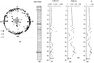Figure 6

To determine the NRM directions, we studied the anisotropy of magnetic susceptibility (AMS). The susceptibility tensor can be represented as an ellipsoid having three main axes: maximum $K_1$, intermediate $K_2$ and minimum $K_3$. Measurements of the magnetic susceptibility of the samples with Multi-Function Kappabridge cappameter (AGICO, Czech Republic) in 15 positions for the AMS assessment showed that the maximum and intermediate axes of the magnetic susceptibility tensor lie in the bedding plane, while the minimum axis is perpendicular to the bedding plane: $K_3 < K_1$, $K_3 < K_2$, where $K_1$ and $K_2$ are $x$ or $y$ components of anisotropy in the plane of the site ($x$ is the direction of the north end of the magnetic needle of the compass) and $K_3$ is normal $(z)$ component. Figure 6 shows the values of the parameters: lineation $L = K_1/K_2$, foliation $F = K_2/K_3$, anisotropy degree $P'$ from the depth of the section. Average values of $L= 1.003$, $F = 1.023$, $P' = 1.028$, shape parameter $T=0.731$ [Tarling and Hrouda, 1993]. Thus, the rock samples have a low planar anisotropy ($\sim 3$%), the shape of the magnetic particles is oblate, which is typical for natural sediments.

The spatial distribution of the directions of the maximum, intermediate, and minimum axes of AMS ellipsoid is shown in Figure 6a. It can be seen from the figure that most rock samples have planar anisotropy characteristic of sedimentary layers. The obtained results indicate that the studied sedimentory rocks are characterized by an undisturbed natural structure.

### Method of Paleomagnetic Research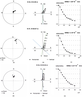Figure 7

With a goal to obtain the directions of magnetization recorded in the sedimentary rocks of the Kop-Takyl section, in this work we used alternating field demagnetization – three samples from each sampling level. Since main magnetic carriers of magnetization in the studied rocks are the monoclinic pyrrhotite and a low concentration of magnetite, low initial values of NRM are typical for the collection samples. To isolate the characteristic component of magnetization, for all samples, the demagnetization curves from the initial NRM$_0$ to 40–80 mT were taken with a step of 2.5–5 mT (Figure 7c). It can be seen in Zijderveld diagrams (Figure 7b) that two components are distinguished. By an alternating magnetic field of $\sim 15-20$ mT, the low-coercitive component of the magnetization is removed. The direction of the low-coercitive component coincides with the direction of the modern magnetic field at the sampling site ($D_{\mathrm{mod.}} = 7.2\mbox{°}$, $I_{\mathrm{mod.}} = 63.4$°). In addition to the viscous component, one component is highlighted on the Zijderveld diagrams, going to zero, the direction of which is taken as the characteristic one.

The value of the secondary magnetization, which is destroyed by the alternating field demagnetization about 20 mT, on average was 40% of NRM$_0$ (Figure 2c). The NRM$_{20}$ value remaining after demagnetization was sufficiently strong with respect to the sensitivity of the magnetometers, which suggested further paleomagnetic studies.

### Results of Paleomagnetic Research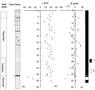Figure 8

The values of the declination $D$ and the inclination $I$ of the natural remanent magnetization obtained after alternating field demagnetization and conducting component analysis according to the program R. J. Enken PMGSC-Paleomagnetism Data Analysis (version 4.2) satisfactorily agree for three samples taken from the same level (the accuracy of the distribution of the directions of the three vectors of natural remanent magnetization is $\sim 275$), which makes it possible to average and plot curves $I$ and $D$ along the section (Figure 8b, Figure 8c). The samples are characterized mainly by positive values of the inclination $I$, with the exception of the horizon located in the lower part of the section at a depth of $\sim 52.12-53.52$ m. Recalculation of the declination and inclination of the characteristic magnetization to the coordinates of the virtual paleomagnetic pole showed that the studied part of the section is characterized by a long interval of normal ($N$) polarity, followed by a short interval of reverse ($R$) polarity at the bottom of the section. To calculate the coordinates of the paleopole, only such angular magnetization elements were used for which the latitude of the virtual paleomagnetic pole exceeded 30°.

During the study, it was found that the considered interval of the Kuvinian beds (Layer 1, $\sim 4$ m) in the lower part is composed of deposits with normal polarity magnetization, and in the upper part rocks with reversal polyrity magnetization are distinguished. The top of the Kuvinian beds and rocks of the Terskian beds are characterized by normal polyrity magnetization. If we don't take into account a single point of indeterminate polarity at the base of the Upper Tarkhanian, then the sediments of the Argunian beds are also normal magnetized.

### Discussion of Results

The difficulties of stratigraphic stratification and differences in the lithology of the Tarkhanian-Chokrakian sections of Eastern Paratethys make it difficult to obtain an uniform paleomagnetic characteristic of these sediments. According to V. M. Trubikhin [Trubikhin, 1998; Voronina et al., 1993], the data obtained as a result of studying a number of sections of the Kerch Peninsula, Eastern Georgia and Turkmenistan, the lower part of the Tarkhanian-Chokrakian interval belongs to the zone of predominantly reverse polarity, the layer of normal magnetized rocks lies higher. Above it there is a low-power zone of reverse polarity, then above again with normal magnetized rocks. Moreover, the reverse polarity zone at the base of the Tarkhanian-Chokrakian interval is correlated with the lower part of the Chron C5Br (15.974–15.160 Ma) and the Lower Langhian of the Middle Miocene of the Mediterranean [Nevesskaya et al., 2004; Trubikhin, 1998]. The presence of reverse magnetized rocks at the base of the Tarhanian is also indicated in the work of E. A. Molostovsky [Molostovsky and Khramov, 1997].

However, there are another points of view. As a result of the studying of the Tarkhanian sediments in Georgia and in Kerch Peninsula (Maly Kamyshlak section) M. A. Pevzner obtained data on the normal polyrity magnetization of the lower part of the Tarkhanian (the Kuvinian, Terskian and lower part of the Argunian beds) [Nevesskaya et al., 2004]. According to M. A. Pevzner [Nevesskaya et al., 2004], these sediments are correlated to the upper part of the Chron C5Cn (16.721–16.268 Ma) and partially to the Lower Miocene.

According to D. Palcu [Palcu et al., 2019] the Tarkhanian sediments in the sections of the Belaya and Psheha rivers of the Western Ciscaucasia have normal polyrity magnetization and related to the Chron C5Bn.1n (14.870–14.725 Ma), corresponding to the middle part of the Middle Miocene Langhian of the Mediterranean.

In this study, it was found that the Kop-Takyl Tarkhanian sediments are characterized by normal polarity magnetization in most of the section. For correct interpretation of the obtained data, in the presence of different interpretations of the position of the Tarkhanian regional stage in the general stratigraphic scale, additional interdisciplinary research is required, including the study of these sediments by cyclostratigraphy methods to determine sedimentation rates and the possible duration of sediment accumulation.

### Conclusions

As a result of petromagnetic and paleomagnetic study of the Tarkhanian sediments of the Miocene of the Kop-Takyl section, it was found that the main carriers of magnetization in the rocks are monoclinic pyrrhotite and a low concentration of magnetite, which leads to low values of natural remanent magnetization. The rocks have an undisturbed natural structure, which suggests the absence of significant secondary transformation of rocks. It is established that the studied interval of the Kuvinian beds in the lower parts is composed of normal magnetized rocks. In its upper part, rocks have reverse polarity magnetization. The rocks of the Terskian beds are distinguished by normal polarity magnetization. If we do not take into account a single point of indeterminate polarity at the base of the Argunian beds, then the sediments of the Upper Tarkhanian are normal magnetized. This conclusion is in a good agreement with the results obtained by D. Palcu for the Tarkhanian sediments in the sections of the Belaya and Psheha rivers of the Western Ciscaucasia [Palcu et al., 2019].

#### Acknowledgments

The authors express their deep gratitude to the researchers of the Geophysical Center of RAS A. Rybkina for comprehensive assistance in organizing field and research work as well as to. A. Odintsova and S. Merkulov for their help in sampling the Kop-Takyl section and preparing the collection for experimental studies. This work was supported by the Russian Science Foundation grant \numero 19-77-10075.

### References

Day, R., M. D. Fuller, V. A. Schmidt (1977) , Hysteresis properties of titanomagnetite: Grain size and composition dependence, Phys. Earth Planet. Inter., 13, p. 260–266, https://doi.org/10.1016/0031-9201(77)90108-X.

Golovina, L. A., I. A. Goncharova (2004) , Plankton and benthos (nannoplankton and bivalves) in the Tarkhan deposits of the Kerch Peninsula and the Western Ciscaucasia, Problems of stratigraphy of the Phanerozoic of Ukraine, p. 147–150, Institute of Geological Sciences, NAS of Ukraine, Kiev (in Russian).

Goncharova, I. A. (1989) , Bivalve Mollusks of the Tarkhan and Chokrak basins, PIN AN USSR, 200 pp., Science, Moscow (in Russian).

Maher, B. A., R. Thompson (eds.) (1999) , Quaternary Climates, Environments and Magnetism, 390 pp., University Press, Cambridge.

Molostovsky, E. A., A. N. Hramov (1997) , Magnetostratigraphy and its Significance in Geology, 179 pp., Publ. Saratov University, Saratov (in Russian).

Neveskaya, L. A., E. I. Kovalenko, E. V. Beluzhenko, et al. (2004) , Explanatory Note to the Unified Regional Stratigraphic Scheme of Neogene Deposits of the Southern Regions of the European Part of Russia, 83 pp., Paleontological Institute of the RAS, Moscow (in Russian).

Palcu, D. V., S. V. Popov, L. A. Golovina, et al. (2019) , The shutdown of an anoxic giant: Magnetostratigraphic dating of the end of the Maikop Sea, Gondwana Research, 67, p. 82–100, https://doi.org/10.1016/j.gr.2018.09.011.

Pan, Y., R. Zhu, J. Shaw, et al. (2001) , Can relative paleointensity be determined from the normalized magnetization of the wind-blown loess of China, Journal of Geoph. Reserch., 106, p. 19,221–19,232, https://doi.org/10.1029/2001JB000360.

Popov, S. V., I. A. Goncharova, T. F. Kozyrenko, et al. (1996) , Excursion guidebook. Neogen stratigraphy and paleontology of the Kerch and Taman Peninsulas, Tethys Programme. Field symposium 4–14 June, 1996, p. 33, PIN, Moscow.

Rostovtseva, Yu. V. (2012) , Sedimentogenesis in the basins of the Middle and Late Miocene of East Paratetis (stratotype Kerch-Taman region), Abstract of the Ph.D. dissertation, 11 Format, Moscow (in Russian).

Tarling, D. H., F. Hrouda (1993) , The Magnetic Anisotropy of Rocks, 277 pp., Chapman &amp; Hall, London.

Taylor, J. (1985) , Introduction to Error Theory, 272 pp., World, Moscow (in Russian).

Trubikhin, V. M. (1998) , Paleomagnetic Scale and Stratigraphy of the Neogene-Quaternary Sediments of Paratetis, Basic Sections of the Neogene of East Paratetis (Taman Peninsula), 13–17 pp., Taman, Volgograd (in Russian).

Voronina, A. A., S. V. Popov, V. M. Trubikhin, V. P. Kalugin (1993) , Aktepinskaya suite of Kopetdag and the position of the border of the Paleogene and Neogene, Stratigraphy, Geological Correlation, 1, no. 3, p. 84–93 (in Russian).

Received 14 April 2020; accepted 5 May 2020; published 29 May 2020.Citation: Pilipenko O. V., E. V. Filina, Yu. V. Rostovtseva, Z. Novruzov (2020), Petromagnetism and paleomagnetism of the Tarkhanian sediments in Kop-Takyl section (the Kerch Peninsula), Russ. J. Earth Sci., 20, ES3003, doi:10.2205/2020ES000712.

Generated from LaTeX source by ELXfinal, v.2.0 software package.